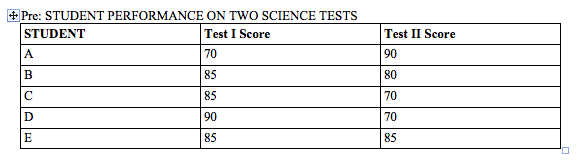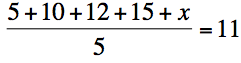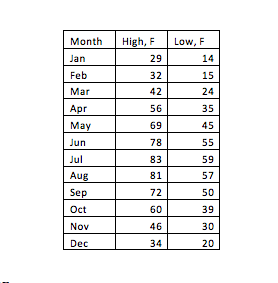# PSAT Math : How to find median

## Example Questions

← Previous 1

### Example Question #1 : MedianThe chart above shows the scores on two tests for five students in a science class. If John's score was equal to the mode for Test I and equal to the median for Test II, then which of the five students represents John's scores?

D

A

E

B

C

B

Explanation:

The mode of a set of numbers is the number that appears most frequently in that set. The median of a set of numbers is the number that appears in the middle when they are arranged in numerical order. For Test I, the mode is 85. When arranged in numerical order, the scores for Test II are:

70, 70, 80, 85, 90.

That makes the median for Test II 80. The only student in the chart with an 85 on Test I and an 80 on Test II is student B.

### Example Question #2 : Median

The average (arithmetic mean) of 1, 7, 9, and n is –1. What is the median of this set?

4

7

–4

–2

1

4

Explanation:

If the mean is –1, then 1 + 7 + 9 + n = –4. Solving for n gives us n = –21. In numeric order, we now have –21, 1, 7, 9. As there is an even number of numbers, we average the middle two. (1 + 7) / 2 = 4.

### Example Question #3 : Median

The average of 5, 10, 12, 15, and x is 11. What is the median?

15

13

12

11

12

Explanation:

You must first find what x is in order to find the median.

To find x, set up the following equation:To solve the equation, first multiply both sides by 5:

5 + 10 + 12 + 15 + x = 55

Then, add up 5 + 10 + 12 + 15 to get 42:

42 + x = 55

x = 13

Now that you know what x is, you are ready to find the median. To find the median, order the numbers from lowest to highest. The median is the number in the middle.

5, 10, 12, 13, 15

### Example Question #4 : MedianThe monthly averaged high-and low-temperatures in Michigan are shown in the given table. What are the median monthly averaged high- and low-temperatures respectively in Michigan?

high: 56F, low: 35F

high: 46F, low: 35F

high: 58F, low: 37F

high: 60F, low: 39F

high: 56F, low: 30F

high: 58F, low: 37F

Explanation:

Median is the middle number when the data are ordered from least to greatest. In this case, there are 12 numbers, so, the median is the average of the middle two numbers, which are the 6th and 7th numbers in an ascending order. For the averaged high-temperature, the median is (56+60)/2=58, and for the averaged low-temperature, the median is (35+39)/2=37

### Example Question #5 : Median

Find the median of the following sequence:

3, 4, 5, 6, 7, 7, 10

7

5

6

10

6

Explanation:

The median is the middle number in a sequence when the numbers are put in order. Since this sequence is already in order from least to greatest, we just need to find the middle number. There are 7 terms so the middle term is (7+1)/2 or the 4th term. This is 6.

### Example Question #6 : Median

Find the median of the data set:

25, 37, 13, 58, 52, 83, 21, 51

13

70

44

83

42.5

44

Explanation:

42.5 is the mean of the data.  13 is the minimum.  83 is the maximum.  70 is the range.

To find the median, list all numbers in order:

13, 21, 25, 37, 51, 52, 58, 83

and find the middle value.  In cases like this where there are two middle numbers (37 and 51), find the mean of these two numbers.

(37+51)/2 = 88/2 = 44

### Example Question #102 : Statistics

The Brenner cousins' heights, in inches, are as follows:

Jeremy: 64

Vanessa: 69

Tracie: 60

Samuel: 70

Raymond: 74

Justin: 72

Patty: 55

Lauren: 52

Keith: 58

What is the median height of the cousins?

58

64

52

60

74

64

Explanation:

To find the median, one must arrange all the heights from the lowest to the highest value and then pick the middle value.

All values: 64 69 60 70 74 72 55 52 58

In order from lowest: 52 55 58 60 64 69 70 72 74

Median: 64

### Example Question #81 : Statistics

Find the median in this set of numbers

2, 100, 52, 97, 1, 7, 22

52

22

7

97

1

22

Explanation:

in order to find the median, arrange the numbers in ascending order, and find the number in the middle of the list

### Example Question #82 : Statistics

Find the median from the given set of numbers

1, 4, 8, 17, 8, 8, 15, 21, 32, 17

15

11.5

7

8

4

11.5

Explanation:

In order to find the median, arrange the numbers from smallest to biggest and find the number in the middle of the set.

In this case, both the 8 and 15 are in the middle of the set; so take the average of those 2 numbers (add both and divide by 2)

### Example Question #83 : Statistics

The number is to be added to the list  {3, 4, 5, 6, 10, 12}. If is an integer, which of the following could be the median of the new list of seven numbers?

I) 5

II) 5.5

III) 6

I and III only

I and II only

I only

I, II, and III

II and III only